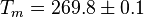# TIP4P/Ice model of water

(Redirected from TIP4P/Ice)

The TIP4P/Ice model  is a re-parameterisation of the TIP4P potential for simulations of ice phases. TIP4P/Ice is a rigid planar model, having a similar geometry to the original Bernal and Fowler model.

## Parameters$r_{\mathrm {OH}}$ (Å)$\angle$HOH , deg$\sigma$ (Å)$\epsilon/k$ (K) q(O) (e) q(H) (e) q(M) (e)$r_{\mathrm {OM}}$ (Å) 0.9572 104.52 3.1668 106.1 0 0.5897 -2q(H) 0.1577

## Virial coefficients

The second virial coefficient has been calculated by Chialvo et al .

## Melting point$T_m = 269.8 \pm 0.1$ K .